# How to use algebra and vectors to simulate airborne objects for your gameIn this tutorial we will learn a bit more about how vectors can be used to simulate the movement of an airborne solid overtime, this will include derivation, integration, vector transformation, forces and acceleration.

# Introduction

In the previous sections, we have learned the basics of vectors (e.g., magnitude, unit vectors, directions, multiplication with scalars, and dot products). We will now, learn a bit more about how vectors can be used to simulate the movement of an airborne solid overtime, this will include:

• Derivation
• Integration
• Vector transformations
• Forces and acceleration

# Prerequisites for the tutorial

• Have completed Part 1
• Be comfortable with using vectors
• An interest in linear algebra

# Length of the tutorial

This tutorial should take 20 minutes to complete

# Objectives of the tutorial

After completing this tutorial you should be able to:

• Understand how Newton’s laws can help to define the trajectory of an object overtime
• Understand the concepts of acceleration, speed, and position, in terms of vectors (i.e., direction and magnitude)
• Understand the difference between integrals and derivatives
• Understand how integrals can help to determine speed and positions, provided that constant forces are applied to a solid overtime

More importantly, you will understand how these relate to your game development as we will see how they can help to do determine the trajectory of an airborne projectile.

Airborne objects can be found in many games; and to make their animation realistic, it is often important to understand and compute animations based on physics’ laws; this can be applied to both 2Dimensiotnal and 3Dimensional objects. For example, in the game illustrated in the next image, each tank (blue and red) need to fire a projectile and destroy the other tank. The trajectory of the projectile will usually depend on the orientation of the canon and the initial speed of the projectile.Image from Blitwise.com

## Introduction

Before we start with the maths, let’s look at three concepts that we will be using in this tutorial: acceleration, speed, and position. These three concepts can be represented as vectors as they have both a value (or intensity) and a direction. The position can be expressed using three coordinates (x,y, and z) and help to locate an object in a three-dimensional space. The speed can also be expressed using three coordinates (x, y, and z) and help to determine in which direction this object is traveling.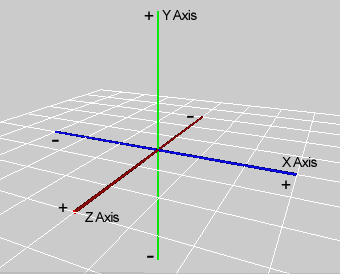Image from stackexchange.com

The speed is a change of position over time: the speed of an object is usually determined by a difference of position over a specific time. For example, moving from the point A (0,0,0) to B (1,1,1) in two seconds, implies a speed of: (A-B)/2 .

In this case the speed is a vector V (1,1,1) = B (1,1,1) – A(0,0,0).Picture from EA.com

The acceleration is a change of speed over time: the speed of an object is usually determined by a difference of position over a specific time. For example, changing speed from V1 (0,0,0) to V2 (0,1,2) in two seconds, implies a speed of: (V2-V1)/2. In car simulations for example, while two cars may be able to achieve great speeds, the car with the greatest capacity for acceleration has an considerable advantage.Image from Gamasutra.com

Both speed and acceleration indicate a change of position or speed, as time changes. In simple terms, we could say that the speed is a derivative (i.e., a change) of position overtime, and that the acceleration is  a derivative (i.e., a change) of speed overtime.

## Derivatives

The term derivatives, although it may sound more complicated than it actually is, indicates how a quantity or variable will change when another (or other) variable(s) change (s). Derivatives, unlike averages, can give the value at a given time and they are usually expressed as follows Derivative of x in respect to t: dx/dt.

So we know that to obtain the speed, we calculate the derivative of the position over time and to obtain the acceleration, we calculate the derivative of the speed over time.

• Derivatives: Position >> Derivation >> Speed >> Derivation >> Acceleration

## Integrals

We know that we can obtain the speed and acceleration of an object using derivatives. But it would be great to know how we could determine the speed from the acceleration or even the position from the speed. This would mean that, if we know the acceleration of a solid, we could in turn determine its speed, and position overtime. To do so, there is a tool that we can use that is called integral, and that does the inverse job as the derivative. For example, while we used a derivative to determine the speed based on the position (i.e., change over time), we can use an integral to determine the position from the speed. In a similar way, we can also use an integral to determine the speed from the acceleration.

• Integrals: Acceleration >> Integration >> Speed >> Integration >> Position

You can  think of derivatives and integrals as a ladder:

• To go up from acceleration to position, we will perform successive integrations
• To go down from position to speed we will perform successive derivationsImage from team-dignitas.com

## Rules for Integrals

As we will be working with integrals, in the next sections, there are some rules that we will be able to use so that we can determine speed and position overtime accordingly.

### Integrating null constants:

To integrate null values, the following formula can be used:In the previous figure, the sign before the zero is the integration sign, and dt means “as time changes” or “over time”. The equation means “The integral of zero overtime is a constant (unknown yet).”. For example, if we had to integrate a null acceleration to find the speed, we will obtain a constant speed. This would make sense, as not accelerating means keeping the speed constant. So far so good :-).

### Integrating constants:

To integrate constant values, the following formula can be used; a constant value is a value that remains the same over time:In the previous figure, the sign at the start of the line  is the integration sign, and dt means “as time changes” or “over time”. This equation means: “The integral of the constant overtime equals this same constant multiplied by time (t), plus another constant c (that is unknown yet).” To use the movement analogy. Let’s say that we move and that a constant speed on the x axis. Our position should then be a linear function of the speed. So if we start at x = 0, and we move towards the positive x-axis at 2 meters per seconds. We will be at x=2 after 1 seconds, x=4 after 2 seconds, x=6 after 3 seconds and so on. So the position will have the form x = 2 * t. But again if we had started at x = 2, we would need to add 2 to this total so that the position over time is x = 2*t + 2. If you compare this equation to the one highlighted above for the integral of a constant, you will notice that they look similar. The only difference is that the constant here equals 2. You may have noticed that I used the term linear function above. If this is unclear to you, or you don’t know the term: let me explain. if you use a 2Dimensional Cartesian referential and use the horizontal axis to represent the time and the vertical axis to represent x, and you plot the points that correspond to t=2*x t = 0 (t=0, x=0), t = 1 (t=1, x=2), or t = 3 (t=3, x=6), you will see that they are on the same line. So t and x, overtime, describe a line; so their relationship is said to be linear.Image from cam.ac.uk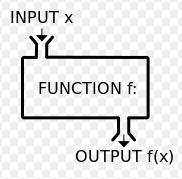Image from Wikipedia.org

You can compare the term function in equations to the term function in programming. When a variable (time) is passed as a parameter to a function of time, the function returns a value based on this parameter. A function is usually noted as f(t), which means the function f takes time as a parameter and returns a corresponding value; or the output of the function f depends on time.

### Integrating the product of a constant by a function of time:

To integrate the product of a constant by a function of time, the following formula can be used:In the previous figure, f(t) means a function of time. In other words an expression for which the result will depend on time.  The equation means “The integral of the product of a constant by a function of time,  overtime,  is this same constant multiplied by the integral of the function overtime.”

## Integrating the sum of two functions

To integrate the sum of two functions of time, the following formula can be used:In the previous figure, f(t) and g(t) are functions of time (in other words, their output will depend on time). The equation means “The integral of the sum of f and g,  overtime,  is the sum of the  integral of the function f overtime, plus the integral of the function g, overtime .”

## Newton to the rescueImage from christinasrateroundup.com

At this stage, we know that if we can identify the acceleration of an object overtime, we can determine, using successive integration (i.e., Integral of (acceleration) = speed and then integral of (speed) = position), the position of our object overtime. So the starting point can be the acceleration. The only remaining question is: how can we determine the acceleration overtime?

Well, you may have heard about Newton and how he determine laws that explained, amongst other thing, gravity. One of his laws specifies that the net force applied to a solid during its trajectory equals its mass multiplied by its acceleration; which can be expressed as follows.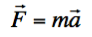OR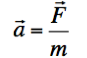So if we know the forces applied to a solid overtime, we can calculate their sum (net force), and then determine the resulting acceleration. Once the acceleration can be identified, as well as the initial conditions of movement (e.g., initial position, speed, and acceleration) the corresponding speed and position can be computed at all times (with successive integrations), hence allowing us to recreate the trajectory of an object over time.

## Determining the trajectory of an airborne object only subject to gravity

In this section, thanks to our knowledge of integrals, we will determine the trajectory of a solid only subject to gravity. For those only interested in the code to simulate this movement, you can jump to the coding section.Let’s consider that the coordinates of the acceleration are (ax, ay, and az) and the coordinates of the net force are (Fx, Fy, and Fz). If we project these forces on the three axes of a Cartesian reference frame (x, y, and z), the components of each vector can be expressed as follows:Decomposing these forces makes it easier to analyze them. The only forces applied to a solid in the air (neglecting air friction and spinning) is its weight; which means that:In the previous equation:

• Line1: The net force F equals the force of gravity.  This force is the mass of the solid multiplied by the gravitational force g.
• Line2: Thanks to Newton’s law we can determine that the net force is the mass of the solid multiplied by its acceleration
• Line3: We combine line 1 and line2
• Line 4: We conclude that the acceleration of the solid is the gravitational acceleration g

Note that g is approximately 9.81 on planet earth; however, it would have a different value on a different planet as it is linked to the radius of the planed and its mass.Image from http://planetcompas.com

Provided that gravity is vertical, the component of the acceleration would be as follows:We also know, based on the previous sections, that the acceleration is a the derivative of the velocity. Inversely, the speed can be obtained by integrating the acceleration. Integration makes it possible to determine the value of a variable from its derivative.

In our case:So the velocity can be determined as follows:In the previous figure, the first line indicates that the speed is the integral of the acceleration overtime and the next lines show this equation on the x-, y-, and z-axes.

Based on the previous equation:In the previous equation: c1, c2, and c3 are constants that we will determine later.

To determine these constants, we will refer to what is called, the initial conditions.Image from caranddriver.com

In other words, we will identify the position, and speed of the object just before its is set in motion, and refer to this time as t = 0; So at t=0 (i.e., time = 0), we know that the object will be at a position P0 (x0, y0, z0) ans have a speed V0 (V0x, V0y, V0z). These initial speed and position can be any value. They just illustrate how this solid was just before it was set in motion. For example, if we consider a ball dropped 2 meters from the ground with no speed, P0 would be (0,2,0) and V0 would be (0,0,0); or, if the ball was thrown in  direction of the positive axis, at 2 meters per second from the ground, P0 could be (0,0,0) and V0 could be (2,0,0).

So at t = 0, we know that the speed is the initial speed V0 (V0x, Voy, V0z). At t=0:

• Vx=V0x
• Vy=V0y
• Vz=V0z

However, based on the equations we have found above, we also know  that:

• Vx = c1
• Vy = -gt+c2 = -g * 0 + c2 = c2 [as t = 0]
• Vz = c3

If we combine the two sets of equations, we obtain, for t = 0:

• Vx = V0x= c1
• Vy = V0y = c2
• Vz = V0z = c3

Therefore:

c1 = V0x; c2=V0y and c3 = V0z.

This, in turn makes it possible to determine Vx, Vy and Vz over time (since we now know the value of c1, c2 and c3):

• Vx (t) = V0x [V is constant on the x axis over time]
• Vy (t) = -gt+V0y
• Vz (t) = V0z [V is constant on the z axis over time]

In Unity, we could simulate this movement as follows.

```var V0: Vector3;
var t:float;
var g = 9.81;
function Start()
{
V0 = transform.position;
}
function Update()
{
t+=Time.deltaTime;
var velocity:Vector3;
velocity.x = V0x;
velocity.y=-g*t+V0y;
velocity.z = V0z;

}
```

At this stage, we know the speed of the solid overtime, and we still need to determine the position. Based on the previous sections, we know that the position can be determined using the integral of the speed over time. So, first, lets recap on the value of the speed overtime. According to the previous equations, the speed can be expressed as follows:

• Vx (t) = V0x
• Vy (t) = -gt+V0y
• Vz (t) = V0z

To determine the coordinates of the position overtime P (Px,Py, Pz), we need to integrate the coordinates of the velocity overtime, this means that: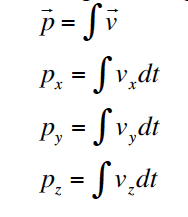Let’s analyze these equations on each axis; on the x-axis:

## Determining Px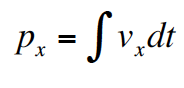Or we know that Vx (t) = Vox; and V0x is a constant; we also know that integrating a constant is the same as multiplying it by t (time) and adding another constant.

So: Px = Vox * t + C1 [where C1 is an unknown constant]

To determine C1, we will, as for previously, look at the initial conditions (just before the movement starts). At t = 0:

• Px = P0x
• Px = V0x * t + C1 = C1 [as t = 0]

Therefore: P0x = C1 or C1 – Pox

## Determining Pz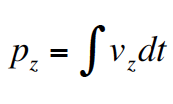We know that Vz (t) = Voz; and V0z is a constant; we also know that integrating a constant is the same as multiplying it by t (time) and adding another constant.

So: Pz = Voz * t + C3 [where C3 is an unknown constant]

To determine C3, we will, as for previously, look at the initial conditions (just before the movement starts). At t = 0:

• Pz = P0z
• Pz = V0z * t + C3 = C3 [as t = 0]

Therefore: P0z = C3 or C3 = P0z

## Determining Py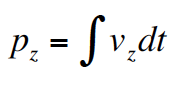We know that Vy (t) = -gt+V0y;

As a result: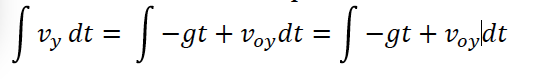At this stage there are two things we can do to simplify this expression:

We know that:

• Integrating the product of a constant by a function of time is the same as multiplying this constant by the integral of the function. In our case, the constant is -g and the function is f(t) = t
• Integrating the sum of two functions is the same as adding the sum of the two integrals.

We can then obtain: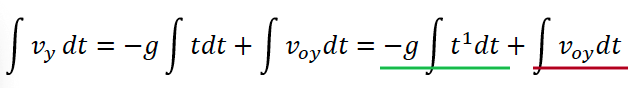In the expression above, we can see that the integral that we are looking for is made of two parts, that we can call A (highlighted in green) and B (highlighted in red).

The second part (in red) is rather easy to calculate as it is related to integrating a constant (V0y), and we have covered this in the previous sections; the result is as follows: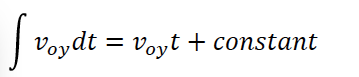For the second part (green), we can notice that it looks like the following: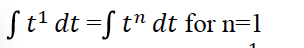This is the same as integrating what we identified as a polynomial early on; and this can be done using the formula: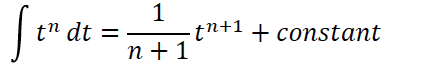So, we can then obtain: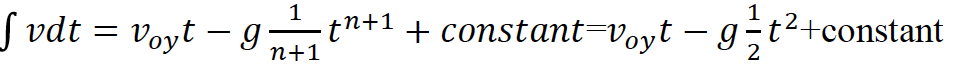If we replace n by 1, this can also be written as follows: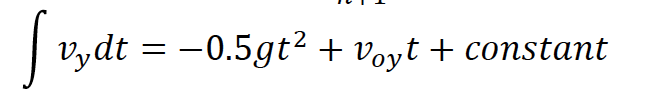To calculate the constant, we need to evaluate the speed position at t=0. At t=0 y=y0 but we also know, based on the previous equation, that at t=0: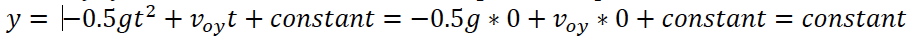So, based on the two previous equations. constant = 0, which mean that: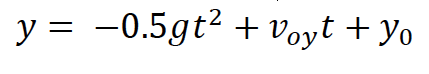The code for the movement would be as follows:

```var V0: Vector3;
var P0: Vector3;

var t:float;
var g = 9.81;
function Start()
{
V0  = new vector3(1,1,1);
P0 = transform.position;
}
function Update()
{
t+=Time.deltaTime;
transform.position.x = V0.x*t + P0.x;
transform.position.z = V0.z*t + P0.z;
transform.position.y = .5*g*t*t V0.y*t + P0.y;

}
```